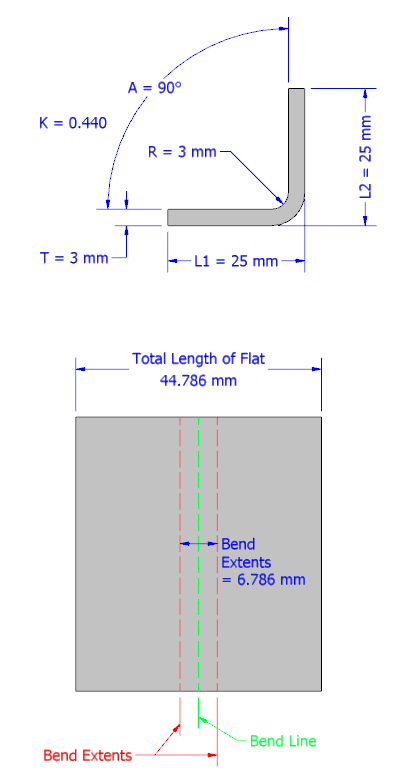## Wednesday, June 15, 2011

### Understanding Bend Allowance and Bend Dedcution in Inventor

Issue:
You want to better understand the difference between Bend Allowance and Bend Deduction and how they relate to Inventor sheet metal parts.Solution:
Bend Allowance and Bend Deduction are not interchangeable terms, so understanding when your parts are using one or the other, or if the parts are using both will help.

Understanding Bend Allowance
Your Inventor sheet metal parts will use a Bend Allowance when you have your unfold method set to use a Kfactor. Kfactors are used as a good approximation of the theoretical percentage of the material thickness at a bend, where it is neither stretched or contracted.

To calculate bend allowance you use a formula that includes a Kfactor. This formula is:

BA = Pi * (A / 180) * (R + K * T)
﻿
Where:
BA = bend allowance
A = bend angle (in degrees)
K = Kfactor
T = material thickness
Pi = 3.14159 (held to 5 decimal places)

So with a test part using these values:
A = 90 degrees
R = 3 mm
K = 0.440
T = 3 mm
Pi = 3.14159 (held to 5 decimal places)
﻿
You'll find BA = 6.786 mm

So if you had a simple model with two legs that were both 25 mm long and if you were to flatten this model you'd find that the total flat pattern length is 44.786 mm:Here is the result of changing the bend angle to 85 degrees, this results in a Bend Allowance of 6.409 mm, and  you'll notice the overall length of the flat pattern is decreased:

Understanding Bend Deductions
Often times we set up a bend table based on data collected from creating test bends on the shop floor using our exact equipment and tooling. Using well created bend table is the most accurate way to create precise sheet metal flat patterns in Inventor, because it uses exact bend deduction numbers.
.
But if you use a bend table for your unfold method instead of a Kfactor you're no longer dealing with a Bend Allowance (calculated from the Kfactor percentage), but instead are using Bend Deductions (which are exact length values to be subtracted from combined leg lengths).

Your flat pattern length can be calculated with this formula:
Total Flat Pattern Length = Leg1 + Leg2 - Bend Deduction

Here is the same part as before, but in this case the bend table specifies a 5 mm bend deduction for 90 degree bends (note this bend deduction is a theoretical value and is not likely to be accurate to what you'd find in the real world):If your bend table does not contain the bend angle used in your part, Inventor uses either an linear extrapolation between two angles that are included in the bend table or it used the specified backup Kfactor if the bend angle falls outside of the included bend angles.

Here is a helpful PDF that discusses calculating Bend Deductions using test bend pieces:
http://mfgcommunity.autodesk.com/files/22201_22300/22265/file_22265.pdf
or here:
http://dl.dropbox.com/u/53790195/Inventor%20Trenches/Sheet%20Metal%20bend%20deduction.pdf